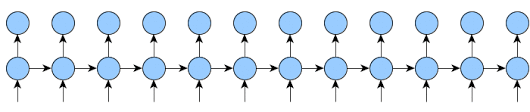# Truncated backprop data clarification

Looking at this post, I wanted to clarify/verify whether or not the `train` method is being called on each sequence.

code from the post:

`````` def train(self, input_sequence, init_state):
states = [(None, init_state)]

outputs = []
targets = []

for i, (inp, target) in enumerate(input_sequence):

state = states[-1].detach()
output, new_state = self.one_step_module(inp, state)

outputs.append(output)
targets.append(target)
while len(outputs) > self.k1:
# Delete stuff that is too old
del outputs
del targets

states.append((state, new_state))
while len(states) > self.k2:
# Delete stuff that is too old
del states
if (i+1)%self.k1 == 0:
# loss = self.loss_module(output, target)

# backprop last module (keep graph only if they ever overlap)
start = time.time()
# loss.backward(retain_graph=self.retain_graph)
for j in range(self.k2-1):

if j < self.k1:
loss = self.loss_module(outputs[-j-1], targets[-j-1])
loss.backward(retain_graph=True)

# if we get all the way back to the "init_state", stop
if states[-j-2] is None:
break
print("bw: {}".format(time.time()-start))
optimizer.step()
``````

If I understand it correctly, the `for` loop is iterating over each item in the sequence, meaning that if `model = self.one_step_module = RNN(...)` , then `self.one_step_module(inp, state)` is only run on a sequence of length 1.

Then the rest of the code runs backprop on the last `k2` items at an interval of `k1` items.

I think that would make sense since, if `inp` was an entire sequence, then we’d be using state from an unrelated example to inform the next example.

is this all correct?

In order to back-propagate you need the `output` and the `target`. Both of them are kept for the last `k1` steps.

``````            outputs.append(output)
targets.append(target)
while len(outputs) > self.k1:
# Delete stuff that is too old
del outputs
del targets
``````

As you may see at the gif I posted, for the rest `(k2-k1)` steps the output is not requered.The `inp` you are referring to is already saved into each `state`, as part of the graph that torch automatically creates and thats why the `k2` last `states` are kept.

``````            states.append((state, new_state))
while len(states) > self.k2:
# Delete stuff that is too old
del states
``````

This implementation do whats exactly denoted on this paper(section 4) as BPTT(h; h’), where h’=k1 and h=k2.